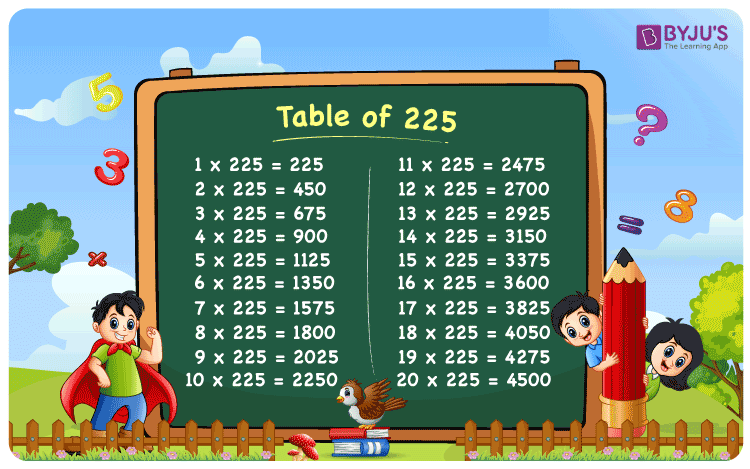Checkout JEE MAINS 2022 Question Paper Analysis : Checkout JEE MAINS 2022 Question Paper Analysis :

# Table of 225

Table of 225 is the multiplication table that includes the multiples of the number, 225. It represents the repeated addition of the number 225. For example, 225 multiplied by 3 is equal to 225 x 3 = 225 + 225 + 225 = 675. Hence, whether we multiply 225 by 3 or add it to itself three times, it will result in same value. 225 times table will help students to solve mathematical calculations quickly. We have also provided a PDF of table 225 to download and learn offline, for free. Let us learn 225-table up to 20 times, in this article.

## Table of 225 Chart

Table of 225 chart is provided here for students to make them learn easily. With the help of this chart, students can solve multiplication related problems easily.## Multiplication Table of 225

In Mathematics, tables play an important role for students to solve arithmetic problems. Since 225 is a greater number and its table is not easy to remember, therefore, we have provided the table of 225 here. This table will represent the multiplication of 225 by consecutive natural numbers up to 20 and the resulting multiple.

### 225 Times Table up to 20

The Maths table of two hundred twenty-five is given below:

 225 x 1 = 225 225 x 2 = 450 225 x 3 = 675 225 x 4 = 900 225 x 5 = 1125 225 x 6 = 1350 225 x 7 = 1575 225 x 8 = 1800 225 x 9 = 2025 225 x 10 = 2250 225 x 11 = 2475 225 x 12 = 2700 225 x 13 = 2925 225 x 14 = 3150 225 x 15 = 3375 225 x 16 = 3600 225 x 17 = 3825 225 x 18 = 4050 225 x 19 = 4275 225 x 20 = 4500

Students can also download the PDF of table 225 for easy access.

## Solved Examples on Table of 225

Q.1: What is the value of 225 multiplied by 4?

Solution: From the table of 225, we have,

225 x 4 = 900

Q.2: Find the value of 225 x 5 + 1200 = ?

Solution: From the table of 225, we have;

225 x 5 + 1200

= 1125 + 1200

= 2325

Q.3: Write 225 as the product of numbers.

Solution: 225 is a whole number. We can prime factorise it to get the product.

Prime factorisation of 225 gives:

225 = 5 × 5 × 3 × 3

Or we can also write it as:

225 = 15 × 15

## Frequently Asked Questions on Table of 225

### What is table of 225?

Table of 225 is the multiplication table that results in multiples when 225 is multiplied by different numbers.

### What is the value of 225 times 8?

225 times 8 is equal to 1800.

### How to Read 225 Table in Words?

Table of 225 is read as:
Two Hundred And Twenty-Five times One is equal to 225
Two Hundred And Twenty-Five times Two is equal to 450
Two Hundred And Twenty-Five times Three is equal to 675
Two Hundred And Twenty-Five times Four is equal to 900
Two Hundred And Twenty-Five times Five is equal to 1125
And so on.

### What should be multiplied by 225 to get 3600?

From the table of 225, when 225 is multiplied by 16 then the product is 3600.